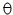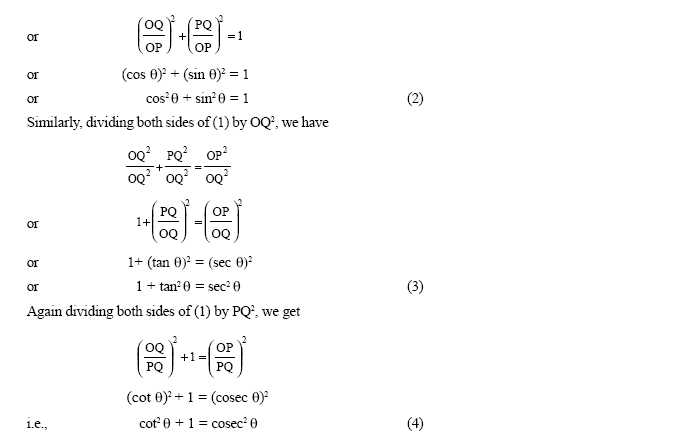Name: ___________________Date:___________________

 Consider any acute angle AOB. P is a point on the ray OB and PQ is perpendicular to OA.We have Sin= PQ/OP Cos= OQ/OP Tan= PQ/OQ Cosec= 1/Sin= OP/PQ Sec= 1/Cos= OP/OQ Cot= 1/tan= OQ/OP Important identities : sin2+ cos2= 1 1 + tan2= sec21 + cot2= cosec2Example: Show that cot+ tan= sec.coseccot+ tan= cos/sin+ sin/cos= cos2+sin2/sincos= 1/sincos= 1/sin. 1/cos= cosec.secDirections: Find the mid point of the segment joining two points and draw a graph for the problems.Name: ___________________Date:___________________